# Math in Focus Grade 5 Chapter 13 Practice 4 Answer Key Triangle Inequalities

This handy Math in Focus Grade 5 Workbook Answer Key Chapter 13 Practice 4 Triangle Inequalities provides detailed solutions for the textbook questions.

## Math in Focus Grade 5 Chapter 13 Practice 4 Answer Key Triangle Inequalities

Complete. Measure the sides of the triangle to the nearest half-inch. Then fill in the blanks.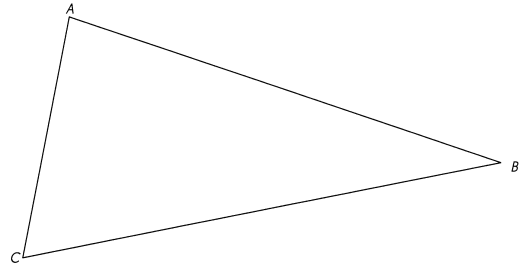Explanation: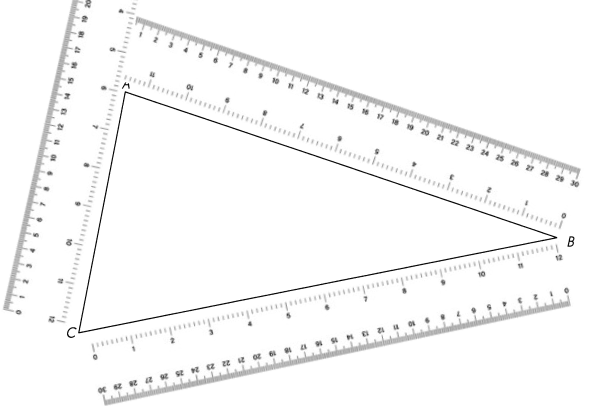Question 1.
AB = _________ in.
AB = 12 in.
Explanation:
With the help of ruler measured the length and updated

Question 2.
BC = _________ in.
BC = 12 in.
Explanation:
With the help of ruler measured the length and updated

Question 3.
AC = ________ in.
AC = 7 in.
Explanation:
With the help of ruler measured the length and updated

Question 4.
AB + BC = ________ in.
AB + BC = 12 + 12 = 24 in.
Explanation:
With the help of ruler measured the length and updated
AB + BC = 12 + 12 = 24 in.

Question 5.
BC + AC = ________ in.
Answer: 12 + 7 = 19 in.
Explanation:
BC + AC =12 + 7 = 19 in.
With the help of ruler measured the length and updated

Question 6.
AB + AC = ________ in.
Answer:12 + 7 = 19 in.
Explanation:
AB + AC = 12 + 7 = 19 in.
With the help of ruler measured the length and updated

Use your answers ¡n Exercises 4 to 6. Fill ¡n the blanks with Yes or No.

Question 7.
Is AB + BC > AC? ______
Explanation:
AB + BC = 12 + 12 = 24
AC= 7
24 > 7

Question 8.
Is BC + AC > AB? ______
Explanation:
BC + AC = 12 + 7 = 19 in.
AB = 12 in.
19 > 7

Question 9.
Is AB + AC > BC? _______
Explanation:
AB + AC = 12 + 7 = 19 in.
BC = 12 in.
19 > 12

Complete. Measure the sides of the triangle to the nearest centimeter. Then fill in the blanks.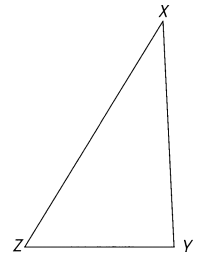Explanation: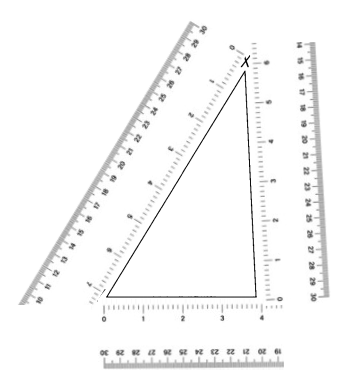Question 10.
XY = ___ cm
XY = 6 cm
Explanation:
With the help of ruler measured the length and updated

Question 11.
YZ = __ cm
YZ = 4 cm
Explanation:
With the help of ruler measured the length and updated

Question 12.
XZ = __ cm
XZ = 7 cm
Explanation:
With the help of ruler measured the length and updated

Question 13.
XY + YZ = __ cm
XY + YZ = 6 + 4 = 10 cm
Explanation:
XY + YZ =6 + 4 = 10 cm
With the help of ruler measured the length and updated

Question 14.
YZ + XZ = __ cm
YZ + XZ = 4 + 7 = 11 cm
Explanation:
YZ + XZ = 4 + 7 = 11 cm
With the help of ruler measured the length and updated

Question 15.
XY + XZ = __ cm
XY + XZ = 6 + 7 = 13 cm
Explanation:
XY + XZ = 6 + 7 = 13 cm
With the help of ruler measured the length and updated

Use your answers in Exercises 10 to 15. Write the sides of the triangle to make the inequalities true.

Question 16.
XY + YZ > ______
XY + YZ > XZ
Explanation:
XY + YZ > XZ
XY + YZ = 6 + 4 = 10 cm
XZ = 7
10 > 7

Question 17.
YZ + XZ > ______
YZ + XZ > XY
Explanation:
YZ + XZ > XY
YZ + XZ = 4 + 7 = 11 cm
XY = 6
11 > 6

Question 18.
XY + XZ > ___
XY + XZ > YZ
Explanation:
XY + XZ > YZ
XY + XZ = 6 + 7 = 13 cm
YZ = 4
13 > 4

Show whether it is possible to form triangles with these lengths.

Question 19.
6 in., 8 in., 12 in.
Yes
Explanation:
6+ 8 > 12
6 + 12 > 8
12 + 8 > 6
sum of two sides should be greater than other side. so its possible to form triangle.

Question 20.
9 in., 13 in., 3 in.
No
Explanation:
9 + 13 > 3
13  + 3  > 9
9  + 3 <   13 – sum of two sides should be greater than other side. so not possible to form triangle.

Question 21.
2 cm, 4 cm, 7 cm
No
Explanation:
2 + 4 < 7
– sum of two sides should be greater than other side. so not possible to form triangle.

The lengths of two sides of each triangle are given. Name a possible length for the third side. The lengths are in whole centimeters or whole inches.

Question 22.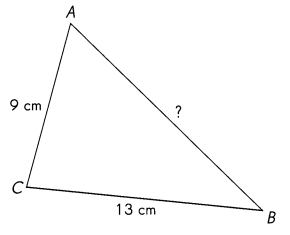AB is greater than 10 centimeters. A possible length for $$\overline{A B}$$ is
____ centimeters.
15 centimeters
Explanation:
the sum of any 2 sides of a triangle must be greater than the measure of the third side.
13 + 9 > 15
13 + 15  > 9
15 + 9 > 13

Question 23.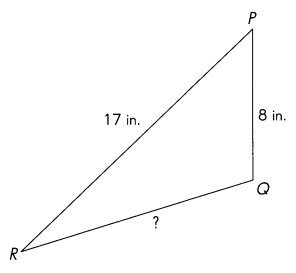QR is greater than 9 inches. A possible length for $$\overline{Q R}$$ is
____________ inches.
11 inches
Explanation:
the sum of any 2 sides of a triangle must be greater than the measure of the third side.
17 + 8 > 11
17 + 11  > 8
8  + 11  > 17

Solve.

Question 24.
In the triangle EFG, EF = 21 centimeters, FG = 11 centimeters. The length of $$\overline{E G}$$ is in whole centimeters and is greater than 25 centimeters. What is a possible length of $$\overline{E G}$$ ?
Answer:  $$\overline{E G}$$  = 26 centimeters
Explanation:
the sum of any 2 sides of a triangle must be greater than the measure of the third side.
21 + 11 > 26
21 + 26  > 11
26  + 11  > 21